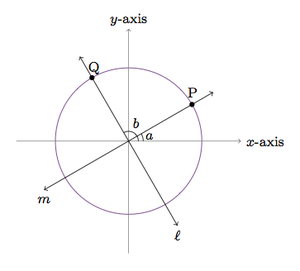# When are two lines perpendicular?

Alignments to Content Standards: G-GPE.B.5

Suppose $\ell$ and $m$ are lines containing $(0,0)$ as pictured below:The unit circle is also shown in the picture as well as two points where the two lines intersect the circle.

1. If $\ell$ and $m$ are perpendicular, what is the relationship between the coordinates of the points labeled $P$ and $Q$?
2. Show that if $\ell$ and $m$ are perpendicular then $$\text{slope}(\ell) = -\frac{1}{\text{slope}(m)}.$$
3. Show that if $\text{slope}(\ell) = -\frac{1}{\text{slope}(m)}$ then $\ell$ and $m$ are perpendicular.
4. What happens to the slope criterion for perpendicular lines $\ell$ and $m$ if one of the lines is vertical?

## IM Commentary

The goal of this task is to examine when two lines in the plane are perpendicular in terms of their slopes. The well known criterion usually reads:

Two lines $\ell$ and $m$ are perpendicular if $\text{slope}(\ell) = -\frac{1}{\text{slope}(m)}.$

Neither $\ell$ nor $m$ can be vertical, in this statement, or else the slope is not well defined; it is true, however, that any vertical line is perpendicular to any horizontal line.

In this task, the two lines are assumed to pass through the origin. In general, a translation can be used to reduce the general case to the one presented here: translations do not impact the slopes of lines and so the argument applies unchanged. If the teacher wishes to see the approach using rotations, presented in the first solution, some encouragement in this direction may be necessary. Students will need to either know or be able to visualize the impact of a 90 degree rotation on point coordinates.

Trigonometric functions provide an additional solution to parts (a) and (b). This approach is more powerful because it allows us to express the coordinates of $P$ and $Q$ whether or not $\ell$ and $m$ are perpendicular: when they are perpendicular, a trigonometric identity gives the desired relationship between the coordinates of $P$ and $Q$. The rigid motion argument used in the first solution is a way of establishing the relevant trigonometric identities.

An additional argument, for part (a) of the second solution, uses the relationship between $\sin$ and $\cos$ of complementary angles (in the case where one of the angles is obtuse and the other has negative measure):

\begin{align} \sin{b} &=\cos{(90-b)} \\ &= \cos{(90-(a+90))} \\ &= \cos{(-a)} \\ &=\cos{a} \end{align}

The first line uses the fact that $b$ and $90-b$ are complementary angles (though neither measures bewteen 0 and 90 degrees). A similar argument applies for the cosine:

\begin{align} \cos{b} &= \sin{(90-b)} \\ &= \sin{(90-(a+90))} \\ &= \sin{(-a)} \\ &= -\sin{a} \end{align}

It is also possible that students have seen these identities before (established in some other way) and can use them here.

## Solutions

Solution: 1 Using Rigid Motions (for parts (a) and (b))

1. If $\ell$ and $m$ are perpendicular then $Q$ is obtained by rotating $P$ by 90 degrees counterclockwise about the origin. This rotation maps the positive $x$-axis to the positive $y$-axis and the positive $y$-axis to the negative $x$-axis. Therefore, if $(a,b)$ are the coordinates of $P$ then $(-b,a)$ are the coordinates of $Q$: graphically, $P$ is $b$ units above the $x$-axis and so $Q$ is $b$ units to the left of the $y$-axis and, similarly, $P$ is $a$ units to the right of the $y$-axis and so $Q$ is $a$ units above the $x$-axis.
2. From our calculation in part (a), the slope of $\ell$ is $\frac{b}{a}$ while the slope of $m$ is $\frac{a}{-b}$. Thus $\text{slope}(\ell) = -\frac{1}{\text{slope}(m)}$ as desired.

3. If $\text{slope}(\ell) = -\frac{1}{\text{slope}(m)}$ this means that the slopes of $\ell$ and $m$ are both non-zero: $\frac{1}{\text{slope}(m)}$ does not make sense if slope($m$) = 0 and the equation cannot be solved if slope($\ell$) = 0. One of the slopes is positive and the other is negative so this means that $\ell$ and $m$ meet in a single point which we call $O$. There is a unique line $k$ containing $O$ and perpendicular to $m$. According to part (b), the slope of $k$ satisfies ${\text{slope}}(k) = -\frac{1}{\text{slope}(m)}$. This means that $k = \ell$ as the two lines have the same slope and both contain $O$. Hence $\ell$ is perpendicular to $m$.
4. The slope of a vertical line is undefined because when finding the change in $y$ over the change in $x$ the latter is always 0. It is true, however, that any vertical line is perpendicular to any horizontal line. Since the slope is well defined except for vertical lines it should always be stipulated, when using the slope criterion, that the lines in question are not horizontal or vertical.

Solution: 2 Using Trigonometric Functions (for parts (a) and (b))

Note that in this solution, the expressions in part (a) for the coordinates of $P$ and $Q$ are valid regardless of whether or not $\ell$ and $m$ are perpendicular so this approach is more general.

1. We first mark the angles made by the points $P$ and $Q$ with the positive $x$-axis:We have

\begin{align} P &= (\cos{a},\sin{a}) \\ Q &= (\cos{b}, \sin{b}). \end{align}
The lines $\ell$ and $m$ are perpendicular so this means that $b = a +90$. We have, as explained in part (a) of the first solution, $\cos{(a+90)} = -\sin{a}$ and $\sin{(a+90)} = \cos{a}$. This means, using our calculations above, that $$Q = (-\sin{a}, \cos{a})$$

2. The slope of $m$ is $\frac{\sin{a}}{\cos{a}}$ and the slope of $\ell$ is $-\frac{\cos{a}}{\sin{a}}$ so ${\text{slope}}(\ell) = -\frac{1}{\text{slope}(m)}$ as desired.